### Mark C. Urban. 2007. The growth–predation risk trade-off under a growing gape-limited predation threat. Ecology 88:2587–2597.

Appendix A. The outcome of model extensions to iteroparity, predators with growth linked to prey capture, prey-size-dependent predator growth, and prey growth plasticity.

(1) Geometric fitness equation for an iteroparous breeder

The general model assumes an annual species. Here, I derive the fitness equations for an iteroparous species that reproduces several times over its lifetime such that each cohort might experience a different predation risk depending on predator community dynamics. The appropriate fitness measure under these assumptions is the geometric mean rate of increase (Bernoulli 1954, Levins 1962, McPeek and Kalisz 1998). The geometric mean rate of increase is calculated depending on the proportion of years in which a gape-limited predator (ρ) occurs compared to those years when a gape-limited predator is absent(1-ρ)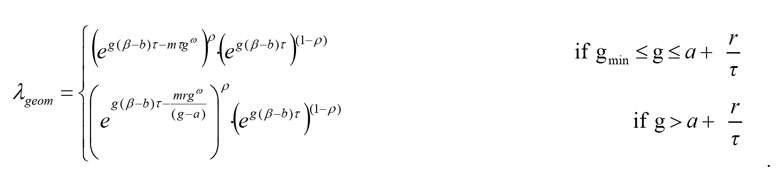(A.1)

Taking the log of fitness and redistributing terms associated with or without gape-limited occurrence (ρ) simplifies the equation considerably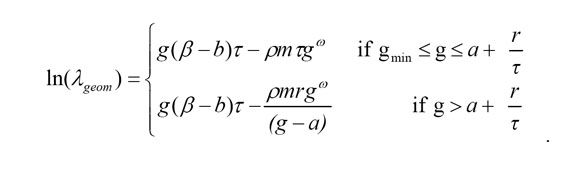(A.2)

Taking the derivative of the log geometric mean rate of increase gives a general equation for prey growth under a growing gape-limited predator: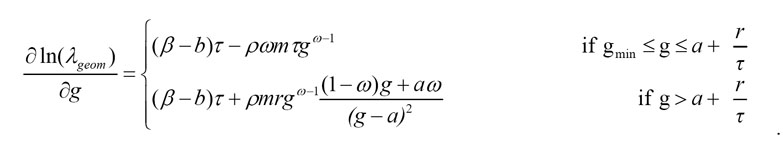(A.3)

The only difference between this model and the model presented in the manuscript is an additional constant (ρ) multiplied to the gape-limited predation risk term.

(2) Survival with a dynamic predator

The general model assumes that a generalist predator grows independently of focal prey mortality. However, a predator’s physiological growth might be dynamically linked to prey growth when the focal prey population makes up a large proportion of its diet. In this case, prey mortality can be translated directly into predator growth scaled by a constant, ε, such that a = εgω. Eq. (5) modified to include a dynamic predator is: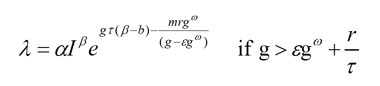(A.4)

The change in log proportional survival with growth is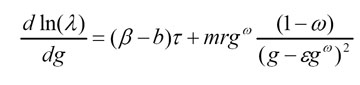(A.5)

Prey growth into a size refuge can be optimal if β > b and ω < 1. This latter constraint on the development of fast growth strategies under a specialist predator is similar to, but slightly more conservative than, conditions selecting for rapid growth under a generalist predator (see main text).

(3) Increasing predation risk with size divergence

General model results assume that predation risk occurs independent of the relative divergence between predator and prey size. However, predation risk might decline with smaller size differences between the predator and the prey if a predator has difficulty handling prey individuals nearly the same size as its gape limitation. Therefore, instead of a fixed mortality risk when outside of the prey size refuge, predation risk could scale proportionately to the positive difference between predator gape size and prey size such that aggregate gape-limited predation risk equals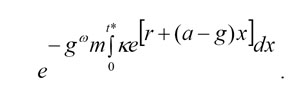(A.6)

In this case, gape-limited predation risk is related to the integral of size differences at each time step x multiplied by a constant (κ). Integration from t* to zero gives: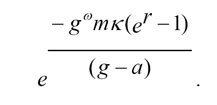(A.7)

Size-proportional predation risk only changes the impact of predation on survival by substituting the positive constant κ(er – 1) for r in the original model. Hence, derivative expectations are not sensitive to the assumed constancy of predation risk with size differences. However nonlinear functions could alter this relationship and suggest a possible future direction for modeling efforts.

(4) Plastic growth rates

So far models have assumed fixed growth strategies such that prey individuals grow at a constant rate after achieving a prey size refuge. Yet, growth can be highly plastic. Many amphibian larvae adjust their growth dependent on the presence of and identity of predator cues presented (Relyea 2003). Here, the general model is altered to include growth plasticity upon entry into prey size refugia. No correlation between early and late growth rates is assumed that might constrain evolution of joint optima (e.g., Via and Lande 1985). The local optimal growth rate (g2*) in the absence of gape-limited predation risk is substituted for the growth rate following attainment of size refuge and solved for optimal early growth until prey size refuge (g1*). Late growth (g2*) is zero if β < b, gmax if β > b and undefined for β = b. However, prey must grow at least as fast as the predator to remain in the size refuge. Therefore, g2* was set, at a minimum, to the predator’s growth rate (a). The modified equation with plasticity is: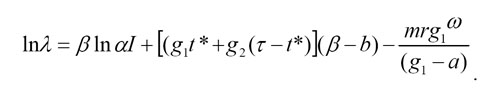(A.8)

The following derivative results by substituting a or gmax for g2: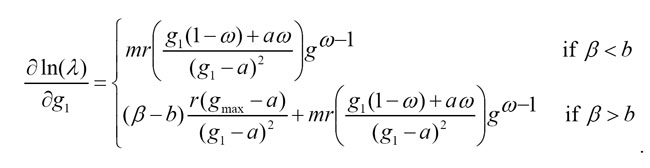(A.9)

All terms are positive in Eq. A.9 when g1(1–ω) + ωa > 0 regardless of the relative magnitude of β vs. b. In addition, under plasticity and unlimited maximum growth rate, no intermediate growth solutions exist. Numerical results mostly confirm this prediction as fast strategies were favored more with plasticity than without plasticity because the risk from gape-unconstrained predators can be lowered by adjusting growth following attainment of size refuge (Fig. A1). However, a small region of intermediate growth rates in Fig. A1 occurs because the assumed gmax is not large enough to support the rapid growth strategy.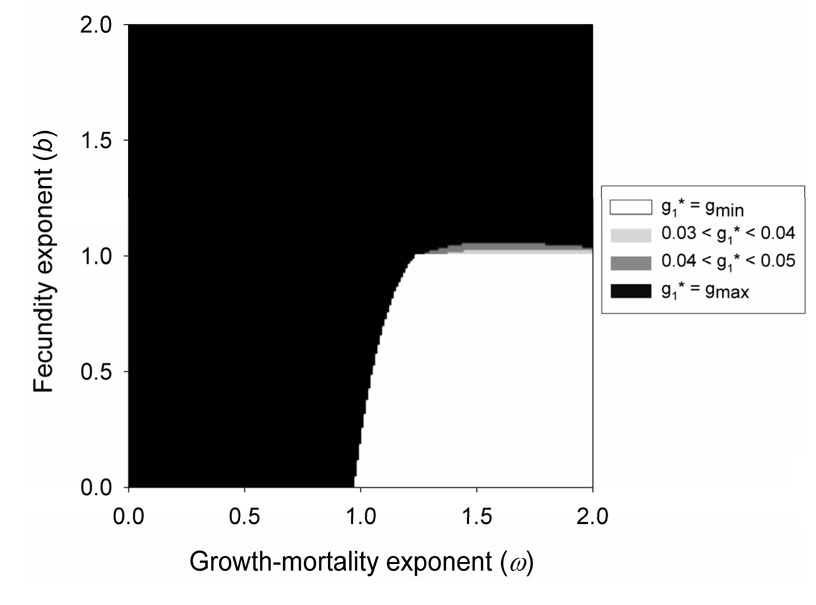FIG. A1. Numerical results for globally optimal growth rates prior to reaching a prey size refuge after allowing for plastic adjustment of growth rate following prey size refuge. The following generic parameters were applied: b = 1; m = 1, τ = 300; a = 0.01; r = ln(2).

LITERATURE CITED

Bernoulli, D. 1954. Exposition of a new theory on the measurement of risk. Econometrica 22:23–36.

Levins, R. 1962. Theory of fitness in a heterogeneous environment. I. The fitness set and adaptive function. American Naturalist 96:361–373.

McPeek, M. A., and S. Kalisz. 1998. On the joint evolution of dispersal and dormancy in metapopulations. Archiv fur Hydrobiologie, Special Issues, Advances in Limnology 52:33–51.

Relyea, R. A. 2003. How prey respond to combined predators: a review and an empirical test. Ecology 84:1827–1839.

Via, S., and R. Lande. 1985. Genotype-environment interaction and the evolution of phenotypic plasticity. Evolution 39:505–522.

[Back toE088-156]